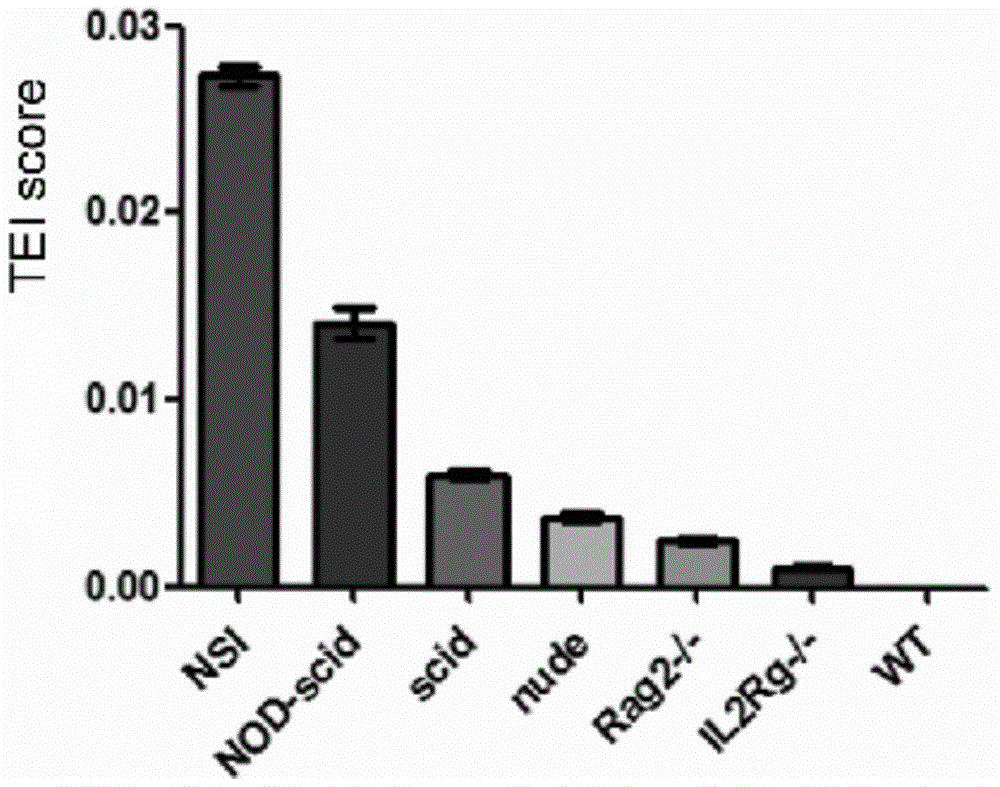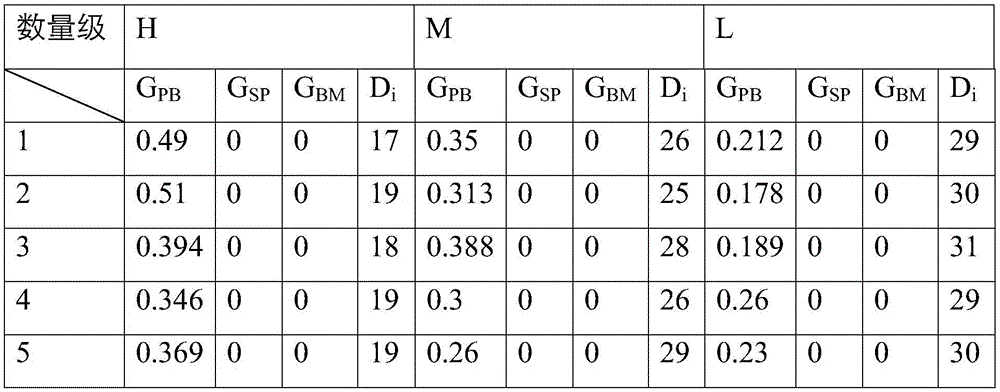# Method for evaluating immunodeficiency degree of immunodeficient mice model

## An immunodeficiency mouse, immunodeficiency technology, applied in animal husbandry, etc., can solve the problems of blind construction and use of immunodeficiency mice, difficulty in the degree of immunodeficiency, unfavorable standardization and promotion of immunodeficiency mice, etc.

Active Publication Date: 2016-10-12
GUANGZHOU INST OF BIOMEDICINE & HEALTH CHINESE ACAD OF SCI
9 Cites 5 Cited by

## AI-Extracted Technical Summary

### Problems solved by technology

However, with the continuous emergence of immunodeficient mice, there is still no standard method for assessing the degree of immunodeficiency in mice. It is difficult to objectively evaluate the degree of immunodeficiency i...
View more

### Method used

 Recognition and killing of the same tumor require more use of the innate immune system, especially NK natural killer cells, and the NK cell defects of IL2rg-/- and Rag2-/- mice are higher, so IL2rg-/- and Rag2 -/- mice's immunodeficiency degree of the same tumor mov...
View more

## Abstract

The invention relates to the field of biology, and discloses a method for evaluating immunodeficiency degree of an immunodeficient mice model. The method comprises: through transplanting neoplastic hematologic disorder cells and solid tumor cells in different orders of magnitudes into an immunodeficient mice model, establishing a tumor mice model, detecting the proportion of tumor cells GPB in internal and external blood of the tumor mice model, the proportion of tumor cells GBM in bone marrow, and the proportion of tumor cells GSP in spleen, according to proportion parameter indexes, evaluating the immunodeficiency degree of the immunodeficient mice model. The method is advantaged in that the method can quantitatively evaluate defect level of the immunodeficient mice, the method relates to simplify and summarize a mathematic model and a calculation method which are obtained through relations between tumor transplanting efficiency and mice immunodeficiency degree, and through scores of immunodeficiency degree, defect difference degree among the immunodeficient mice can be compared. The method is simple and feasible, and is easy to operate, and the method has good application prospect.

Application Domain

Animal husbandry

Technology Topic

Bone marrowHematologic disorders +13

## Image

•••## Examples

• Experimental program(8)

### Example Embodiment

 Example 1: Specific implementation steps:
 Construction of a human hematoma cell line with marker genes
 In order to facilitate the promotion and application of the invention, the human chronic myeloid leukemia cell line K562 is used as the standard (xenogeneic) human blood tumor cells for the immunodeficiency mathematical model, and the human lung adenocarcinoma cell line A549 is used as the standard for the immunodeficiency mathematical model ( Xenogeneic) human solid tumor cells, and introduce marker genes (such as GFP green fluorescent gene, Tdtomato red fluorescent gene, Luciferase luciferase, etc.) into the K562 cell line. The following describes the GFP green fluorescent gene transfection method, packaging Lenti-GFP virus to infect tumor cell lines, expanding the infected cells, sorting successfully infected GFP-positive tumor cells, and obtaining GFP-positive cells with a purity of greater than 98%.
 Construction of Hematoma Mouse Model and HTEI Calculation
 Of different orders of magnitude (10 6 (H), 10 5 (M), 10 4 (L)) Hematoma cell line (K562-GFP) with marker gene is transplanted into experimental mice of specific immunodeficiency strain to be evaluated (5 experimental mice are used for transplantation of each order of magnitude). After transplanting tumor cells, observe the mice every day Rat status. When the mouse is sick, record the survival time of the mouse (D i ) And the proportion of tumor cells (GFP-positive cells) in the blood, spleen, and bone marrow of mice (G PB , G SP , G BM ), using the following formula to calculate the HTEI(H), HTEI(M), HTEI(L) of each order of magnitude,
 Gi is G PB , G BM , G SP The sum, it should be noted that Gi, D i All are the average values ​​obtained from multiple parallel repeated experiments. In this example, 5 mice are used in parallel repeated experiments 5 times, and finally obtained, and then the average is calculated according to HTEI(H), HTEI(M), HTEI(L) HTEI;
 Construction of solid tumor mouse model and calculation of STEI
 Porting different orders of magnitude (10 6 (H), 10 5 (M), 10 4 (L)) Solid tumor cell line (A549 is transplanted into experimental mice of specific immunodeficiency strains to be evaluated (5 experimental mice are used for transplantation of each order of magnitude). After transplantation of tumor cells, the status of the mice is observed every day. Human solid tumors are transplanted After a certain period of time for the cell line (different tumor cell lines have different observation times, the A549 cell line is observed and recorded 30 days after transplantation), weigh and record the mass of the solid tumor formed (Wn, unit: g), and set D n =30 and Wn are substituted into the formula, Calculate STEI(H), STEI(M), STEI(L), and finally calculate the average STEI.
 Assess the degree of immunodeficiency of this strain of mice
 Put the average HTEI and average STEI calculated in the previous steps into the formula Finally, the total tumor action index TEI of this strain of immunodeficiency mouse model was calculated, and the index was negatively correlated with the degree of immunodeficiency.
 The higher the TEI value, the more severe the immunodeficiency of the strain of mice; the lower the TEI value, the more complete the immune system of the strain of mice. The method described above is a comprehensive assessment of the degree of immunodeficiency in mice. For specific immune system conditions, such as the number and function of T cells or B cells or NK cells, and the degree of immune system deficiency against HIV infection, TEI can only provide Reference role
 For the assessment of specific immune system conditions, the mathematical model described in the above formula can also be used to evaluate the animal model. It only needs to make certain modifications to the experimental settings such as tumor transplantation experiment, tumor cells, transplantation magnitude, and obtaining evaluation parameters. That's it.

### Example Embodiment

 Embodiment two:
 Using the method for evaluating the degree of immunity in mice of the present invention, comprehensively evaluate the degree of immunodeficiency (TEI) of NSI (NOD-scid IL2rg-/-) mice. NSI ),Specific steps are as follows:
 1.1.HTEI NSI Numerical calculation: transplant H, M, and L K562-GFP cells into NSI mice. Observe the mice every day. Obtain and record the experimental parameters when the mice develop disease as follows:
 Table 1. Parameters of NSI mouse-human hematological tumor transplantation model

 Substituting the above parameters into the formula:
 HETI ( N S I , H ) = 1 5 X n = 1 5 G n D i = 0.023
 HETI ( N S I , M ) = 1 5 X n = 1 5 G n D i = 0.012
 HETI ( N S I , L ) = 1 5 X n = 1 5 G n D i = 0.007
 HTEI NSI =average(HTEI (NSI, H) , HTEI (NSI, M) , TEI (NSI, L) )=0.014
 1.2.STEI NSI Numerical calculation: transplant A549-GFP cells of the order of H, M, and L into NSI mice. Observe the mice every day. Obtain and record the experimental parameters when the mice develop disease as follows:
 Table 2. Parameters of NSI mouse-human solid tumor transplantation model

 Substituting the above parameters into the formula:
 STEI ( N S I , H ) = 1 5 X n = 1 5 W n D n = 0.059
 STEI ( N S I , M ) = 1 5 X n = 1 5 W n D n = 0.051
 STEI ( N S I , L ) = 1 5 X n = 1 5 W n D n = 0.012
 STEI NSI =average(STEI (NSI, H) , STEI (NSI, M) , STEI (NSI, L) )=0.040
 1.3. Evaluation of the degree of immunodeficiency in NSI mice, TEI NSI Numeral Calculations:
 HTEI obtained above NSI And STEI NSI Substitute the value of into the formula to get
 TEI s t r a i n = HTEI N S I + STEI N S I 2 = 0.027
 From the above, the immunodeficiency score of NSI mice is 0.027.

### Example Embodiment

 Example three
 Using the method for evaluating the degree of immunity of mice of the present invention, comprehensively evaluate the degree of immunodeficiency (TEI) of NOD-scid (abbreviation: NS) mice NS ),Specific steps are as follows:
 1.4.HTEI NS Numerical calculation: transplant K562-GFP cells of the order of H, M, and L into NOD-scid mice, observe the mice every day, obtain and record the experimental parameters when the mice develop disease as follows:
 Table 3. NOD-scid mouse-human hematological tumor transplantation model parameter table

 Substituting the above parameters into the formula:
 HETI ( N S , H ) = 1 5 X n = 1 5 G n D i = 0.012
 HETI ( N S , M ) = 1 5 X n = 1 5 G n D i = 0.003
 HETI ( N S , L ) = 1 5 X n = 1 5 G n D i = 0.000
 HTEI NS =average(HTEI (NS, H) ), HTEI (NS, M) , TEI (NS, L) ) = 0.009
 1.5.STEI NS Numerical calculation: transplant A549-GFP cells of the order of H, M, and L into NOD-scid mice. Observe the mice every day. Obtain and record the experimental parameters when the mice develop disease as follows:
 Table 4. NOD-scid mouse-human solid tumor transplantation model parameter table

 Substituting the above parameters into the formula:
 STEI ( N S , H ) = 1 5 X n = 1 5 W n D n = 0.050
 STEI ( N S , M ) = 1 5 X n = 1 5 W n D n = 0.020
 STEI ( N S , L ) = 1 5 X n = 1 5 W n D n = 0.000
 STEI NS =average(STEI (NS, H) , STEI (NS, M) , STEI (NS, L) )=0.023
 1.6. Evaluation of immunodeficiency in NOD-scid mice, TEI NS Numeral Calculations:
 HTEI obtained above NS And STEI NS Substituting the value of into the formula, we get
 TEI N S = HTEI N S + STEI N S 2 = 0.016
 From the above, the immunodeficiency score of NOD-scid mice is 0.016.

## PUM## Description & Claims & Application Information

We can also present the details of the Description, Claims and Application information to help users get a comprehensive understanding of the technical details of the patent, such as background art, summary of invention, brief description of drawings, description of embodiments, and other original content. On the other hand, users can also determine the specific scope of protection of the technology through the list of claims; as well as understand the changes in the life cycle of the technology with the presentation of the patent timeline. Login to view more.
Who we serve
• R&D Engineer
• R&D Manager
• IP Professional
Why Eureka